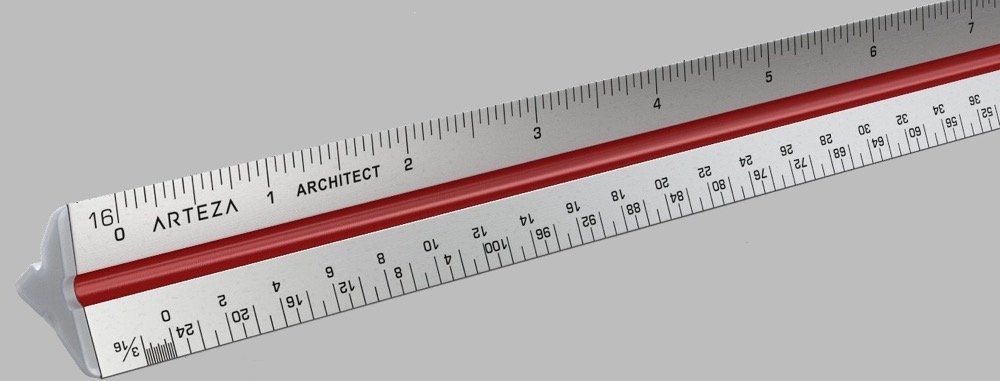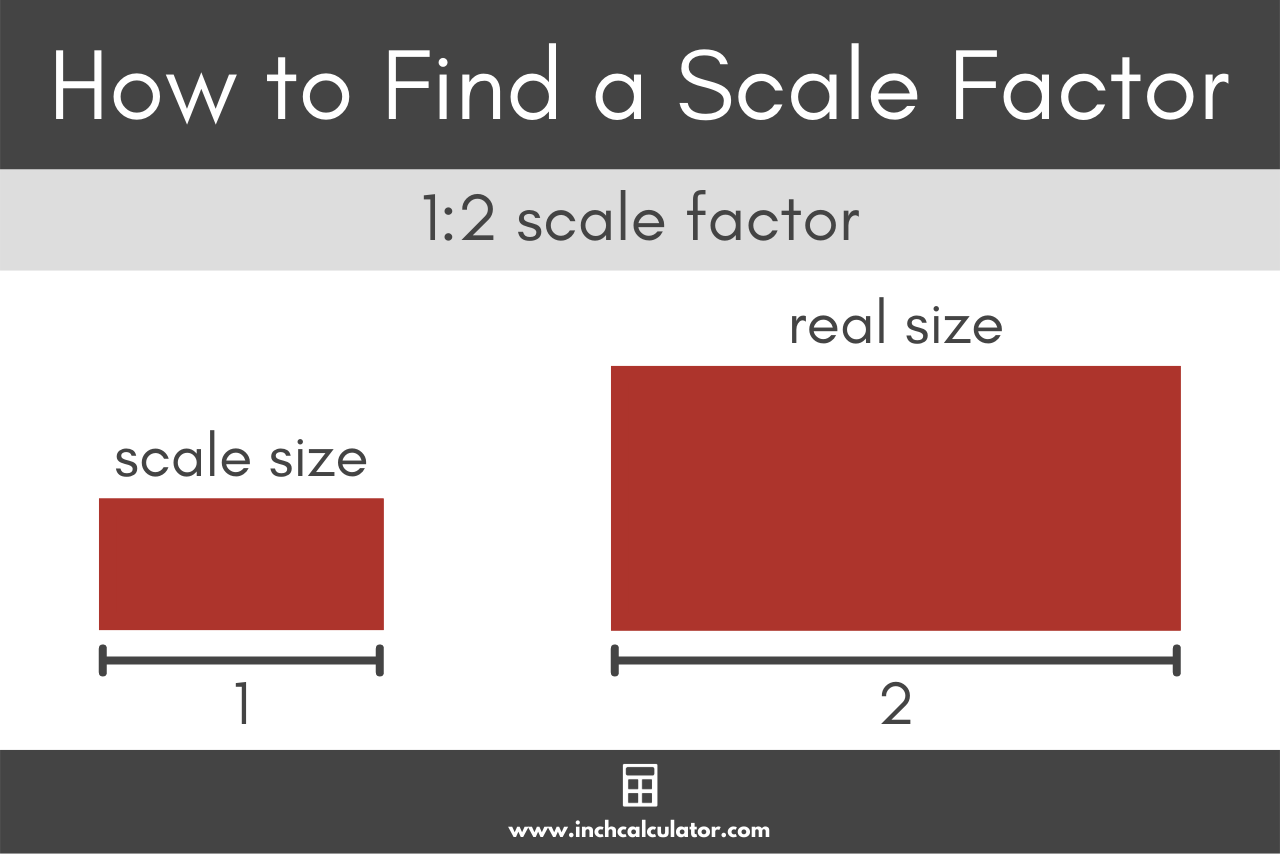# Scale Conversion Calculator & Scale Factor Calculator

Scale a measurement to a larger or smaller measurement, which is useful for architecture, modeling, and other projects. You can also add the real size and scaled size to find the scale factor.

Scale Factor:
:

Scale Factor:
:

## Scale Size:

4

### Scale Factor

1:8
Learn how we calculated this below

## How to Scale a Measurement

Making a measurement smaller or larger, known as scale conversion, requires a common scale factor, which you can use to multiply or divide all measurements.

To scale a measurement down to a smaller value, for instance, when making a blueprint, simply divide the real measurement by the scale factor. The scale factor is commonly expressed as 1:n or 1/n, where n is the factor.

For example, if the scale factor is 1:8 and the real measurement is 32, divide 32 ÷ 8 = 4 to convert.

To convert a smaller, scaled measurement up to the actual measurement, simply multiply the scaled measurement by the scale factor. For example, if the scale factor is 1:8 and the scaled length is 4, multiply 4 × 8 = 32 to convert it to the larger actual size.A common tool used to scale a measurement from a real-world measurement is an architect’s scale rule. These are often used for scaling drawings and blueprints for buildings.

There are also engineering rules that are primarily used in civil engineering to scale measurements for roadways and land development. For example, when creating detail drawings, a scale of 1:10 is often used, and when creating working plans, scales of 1:20 or 1:40 are the preferred choices.

## How to Find the Scale Factor

A scale factor is a ratio of two corresponding measurements or lengths. You can use the factor to increase or decrease the dimensions of a geometric shape, drawing, or model to different sizes. You can find the scale factor in a few easy steps.### Step One: Use the Scale Factor Formula

Since the scale factor is a ratio, the first step to finding it is to use the following formula:

scale factor = scaled size / real size

So, the scale factor is a ratio of the scaled size to the real size.

### Step Two: Simplify the Fraction

The next step is to reduce or simplify the fraction.

If you’re scaling down, that is, if the scaled size is smaller than the actual size, then the ratio should be shown with a numerator of 1. If you’re scaling up, that is, if the scaled size is larger than the actual size, then the ratio should be shown with a denominator of 1.

To find the final scale factor when you’re scaling up, reduce the ratio to a fraction with a denominator 1. To do this, divide both the numerator and the denominator by the denominator.

Note: by doing this, the numerator may become a decimal. This may or may not be desired, depending on your use case. If it’s not desired, then simply reduce the fraction like you would normally.

If you’re scaling down, then reduce the fraction so that the numerator is 1. You can do this by dividing both the numerator and the denominator by the numerator. Again, this may not result in whole numbers, so adjust accordingly.

Our fraction simplifier can help with this step if needed.

### Step Three: Rewrite the Fraction as a Ratio

Finally, rewrite the fraction as a ratio by replacing the fraction bar with a colon. For instance, a scale factor of 1/10 can be rewritten as 1:10.

For example, let’s find the scale factor used on an architectural drawing where ½” on the drawing represents 12″ on the final building.

Begin by replacing the values in the formula above.

scale factor = ½” / 12″

Since the drawing is scaled down, then the scale factor should be reduced to a fraction with a numerator of 1.

Multiply both the numerator and denominator by 2 to simplify.

scale factor = ½” × 2 / 12″ × 2 = 1 / 24

And finally, rewrite the fraction as a ratio.

scale factor = 1 / 24 = 1:24

Thus the scale factor for this drawing is 1:24.

## How to Reduce the Scale Factor

If you already know the scale factor, but it is not in the form of 1:n or 1/n, then some additional work is needed to reduce or simplify it. If the ratio is 2:3, for example, then you’ll need to reduce it so that the numerator is 1.

Use our ratio calculator to reduce a ratio. You can also reduce a ratio by dividing both the numerator and the denominator by the numerator.

For example: reduce 2/3 by dividing both numbers by 2, which would be 1/1.5 or 1:1.5.

2 ÷ 2 = 1
3 ÷ 2 = 1.5
scale factor = 1:1.5

## Architectural Scales

Architectural scales often relate a measurement, in feet, of a building to inches on a drawing. You can quickly find the scale factor for an architectural scale by inverting the fraction, then multiplying it by 12 (inches/foot).

For example, to find the scale factor on a drawing equaling 1′ on a building (1/16″ = 1′), start by inverting the fraction 1/16 so that it becomes 16/1. Then, multiply that by 12, which is 192. So, the scale factor for 1/16″ = 1′ is 1:192.

Scale factors for common architectural scales
Drawing Scale Scale Factor
1/16″ = 1′ 1:192
3/32″ = 1′ 1:128
1/8″ = 1′ 1:96
3/16″ = 1′ 1:64
1/4″ = 1′ 1:48
3/8″ = 1′ 1:32
1/2″ = 1′ 1:24
3/4″ = 1′ 1:16
1″ = 1′ 1:12
1 1/2″ = 1′ 1:8
3″ = 1′ 1:4

## Engineering Scales

Engineering scales are represented in the same units for both the drawing and the actual measurement. For example, if you have a ratio of inches on a drawing to feet in reality, you can quickly find the scale factor for an engineering, or civic, scale by multiplying the feet portion by 12 (inches/foot).

As an example, to find the scale factor for 1″ = 30′, multiply 30′ by 12, which is 360. So, the scale factor for 1″ = 30′ is 1:360.

Scale factors for common engineering scales
Drawing Scale Scale Factor
1″ = 10′ 1:120
1″ = 20′ 1:240
1″ = 30′ 1:360
1″ = 40′ 1:480
1″ = 50′ 1:600
1″ = 60′ 1:720
1″ = 70′ 1:840
1″ = 80′ 1:960
1″ = 90′ 1:1080
1″ = 100′ 1:1200

## Common Model Scales

This table lists some common scale factors you may come across when dealing with different types of models.

Common scale factors used for models and hobbies
Scale Factor Model Type
1:4 steam trains, RC planes
1:8 steam trains, cars
1:10 figures
1:12 cars, motorcycles, dollhouses
1:16 steam trains, cars, motorcycles, military vehicles, figures
1:18 diecast cars
1:20 formula one cars
1:22.5 G-gauge trains
1:24 cars, trucks, aircraft, dollhouses
1:25 cars, trucks
1:32 1 gauge trains, cars, aircraft, figures
1:35 military vehicles
1:43 O-gauge trains, cars, trucks
1:48 O-gauge trains, dollhouses, Lego minifig
1:64 S-gauge trains, diecast cars, Hotwheels/Matchbox
1:72 aircraft, military vehicles, boats, cars
1:76 aircraft, military vehicles
1:87 HO-gauge trains, military vehicles
1:96 ships, spacecraft
1:100 aircraft, spacecraft
1:120 TT-gauge trains
1:144 ships, rockets, spacecraft
1:160 N-gauge trains, wargaming
1:200 aircraft, ships
1:220 Z-gauge trains
1:285 wargaming
1:350 ships
1:700 ships
1:720 ships

### Is scale factor a fraction?

Yes, the scale factor can be represented as a fraction that describes the relative size between a model or drawing, and the actual object.

### Is scale factor always greater than 1?

No, the scale factor is not always greater than one. It will be greater than one if the model is smaller than the actual object, but smaller than one if the model is larger than the actual object.

Typically we make models that are smaller than the object being modeled, hence why the scale factor is usually greater than one.

### How do you calculate scale from a drawing?

You can calculate scale from a drawing by measuring what length on the drawing corresponds to what length on the actual object. For example, if 1 inch on the drawing equals 3 inches on the actual object, then the scale is 1:3.

### What is an example of a scale factor?

An example of a scale factor is the ratio used on a model airplane to describe how much bigger the actual airplane is than the model. If the actual airplane is ten times bigger than the model, then the scale factor is 1:10.